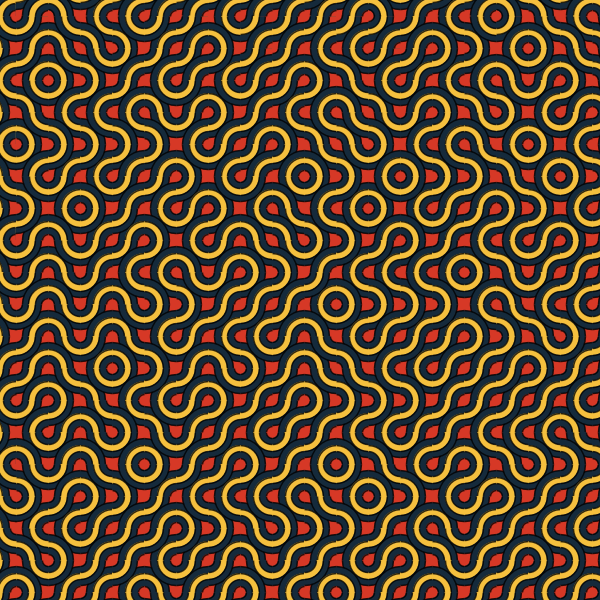I found this P5*.js sketch on openprocessing https://www.openprocessing.org/user/23616/ by Manoylov AC which was in need of some serious refactoring, here is my JRubyArt version (javascript is just horrible) which is much shorter and a bit drier.

``````attr_reader :cols, :coloured

PALETTE = %w[#152A3B #158ca7 #F5C03E #D63826 #0F4155 #7ec873 #4B3331].freeze

def settings
size(600, 600)
end

def setup
background 0
sketch_title 'Arc Pattern'
# create a java primitive array of signed int
@cols = web_to_color_array(PALETTE)
stroke_weight 1.5
stroke_cap SQUARE
stroke(0, 200)
@coloured = true
end

def draw
arc_pattern
end

def sep_index(idx, length)
(idx - (length - 1) * 0.5).abs.floor
end

def sep_color(idx, number)
cols[sep_index(idx - 1, number + 1)]
end

def arc_pattern
circ_number = rand(4..10)
block_size = rand(30..70)
back_color = coloured ? cols.last : 255
fill(back_color)
rect(0, 0, width, height)
half_block = block_size / 2
two_block = 2 * block_size
grid(width + two_block, height + two_block, block_size, block_size) do |x, y|
push_matrix
translate x, y
rotate HALF_PI * rand(1..4)
circ_number.downto(0) do |i|
diam = two_block * i / (circ_number + 1)
ccolor = i < 2 || !coloured ? back_color : sep_color(i, circ_number)
fill ccolor
arc(-half_block, -half_block, diam, diam, 0, HALF_PI)
arc(half_block, half_block, diam, diam, PI, PI + HALF_PI)
end
pop_matrix
end
no_loop
end

def mouse_pressed
@cols = shuffle_array(cols) if coloured
loop
end

def key_typed
case key
when 'c', 'C'
@coloured = !coloured
when 's', 'S'
save(data_path('arc_pattern.png'))
end
end

# do a ruby shuffle! on a primitive java array
def shuffle_array(ar)
cols = ar.to_a
cols.shuffle!
cols.to_java(Java::int)
end
``````